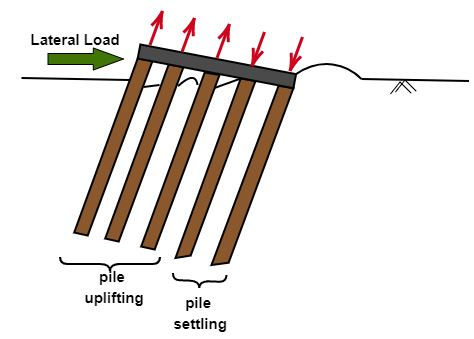top of page
Search
• APSEd

In the design of pile foundation, lateral loads like wind load, seismic force, earth pressure in retaining walls, etc are considered.

As per conventional approach of pile foundation design, it was assumed that vertical piles cannot resist lateral loads whereas only inclined piles can resist lateral loads.But later methods were developed which explained lateral loading of vertical piles and they are mainly grouped into 2 main categories:

1. Based on the plastic theory

2. Based on elastic theory

Here, I will discuss in detail about the method based on an elastic theory using the concept of subgrade modulus as it is widely used. It is the Reese, Matlock and Davisson method.

Reese and Matlock proved that soil modulus varies linearly with depth of pile for cohesionless and normally consolidated cohesive soils and it remains constant for overconsolidated clays.

Now let's see in detail about the Reese and Matlock approach for analysis of laterally loaded piles.

## Reese and Matlock approach

Reese and Matlock gave a formula for relative stiffness factor, T for long piles having L/T>5 where L is the pile depth. The formula of T goes as below-

T = (EI/Nh)^(1/5)

Where E and I are the moduli of elasticity and moment of inertia of pile material respectively

Nh is the unit modulus of subgrade reaction of soil

When a vertical pile of length L subjected to a lateral load Qg and moment Mg at the ground level, the solution for horizontal deflection, y of the pile at any depth is given by-

y = f(x, T, L, K, EI, Qg, Mg)

Here, y = yA+yB

yA: deflection due to Qg

yB: deflection due to MgBased on this method various non-dimensional parameters have been defined while giving the solution as given below.

• Depth coefficient, Z = x/T (Maximum depth coefficient Zmax = L/T)

• Soil modulus function, Φ(Z) = KT^4 / EI

• Deflection coefficient for Qg, Ay = [yA x (EI)] / [Qg x T^3]

• Deflection coefficient for Mg, By = [yB x (EI)] / [Mg x T^2]

The solutions of the equation for deflection are expressed as shown:

Deflection, y = yA + yB = (Qg x T^3 / EI) x Ay + (Mg x T^2 / EI) x By

Slope, s = sA + sB = (Qg x T^2 / EI) x As + (Mg x T / EI) x Bs

Where, As and Bs are slope coefficients

Moment, m = mA + mB = (QgT) x Am + MgBm

Where Am and Bm are moment coefficients

Shear, v = vA + vB = QgAv + (Mg/T) x Bv

Where Av and Bv are shear coefficients

Soil reaction, p = pA + pB = (Qg/T)Ap + (Mg/T^2)Bp

Where Ap and Bp are soil reaction coefficients

• If the pile is fixed to the pile cap, the fixity provided at the pile head is equivalent to a moment Mg = -0.93QgT at the ground level

• For a free-head pile, Mg due to the fixity of the pile is zero.

• For piles with intermediate fixity, interpolation is done.

This is all you need for laterally loaded piles, try to answer this simple question.

### Try this out!

Subscribe to APSEd Blog and get to know the latest.